# 4D Visualization

## Projections (1)

Projection is a method of representing an N-dimensional object using only (N-1) dimensions. There are several different methods of projection, but they all have the same underlying idea: imaginary rays called projectors are emanated from the object towards an (N-1)-dimensional projection plane. The intersections of these rays with the projection plane produce an image of the object. This is like taking a picture with a camera: light from the object travels in straight lines (rays) and strike the film (the projection plane), producing an image (the photograph) of the object.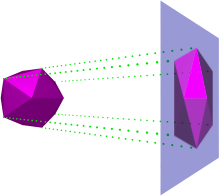The above figure illustrates this process. The object is an icosahedron, residing in 3D space. The green dotted lines show some of the projectors, mapping points of the icosahedron to the projection plane. The resulting 2D image is a “photograph” of the icosahedron.

Projections are an easier method of exploring higher dimensions, because it gives us an integrated view of the object, rather than isolated bits of information such as in the cross-section method. In fact, our own eyes work this way: the retina captures a projection of a 3D object outside of us, producing a 2D image which we then reconstruct into a 3D model in our mind. The image captured this way retains valuable information about the 3D object: features such as corners, edges, and the shape and number of faces, are represented as an integrated whole. Consider the following projection of the cube: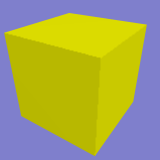We can immediately see where the corners of the cube are, and that its faces are tetragonal. We can see that each corner has three faces meeting at it. Now look again at the sequence of cross-sections we saw in the previous chapter: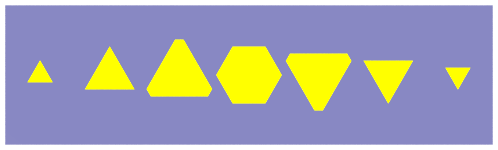Can you pick out where the corners of the cube are in these cross-sections? What about the shape of the cube's faces? How many faces meet at each corner? We would have to analyse these cross sections very carefully in order to derive this information. However, using projections this information is immediately available. Although the cross-section method does give us valuable information, the projection method is much easier to understand.

### Types of Projections

There are two main categories of projections: parallel projection and perspective projection. In parallel projection, the projection rays are parallel to each other. In perspective projection, the rays converge on a single point behind the projection plane. Both types of projection are useful in examining higher-dimensional objects.

### Parallel Projection

Parallel projection may be further classified into orthographic projection, where the rays are always perpendicular to the projection plane, and oblique projection, where the rays intersect the projection plane at an angle.

For example, here is a 3D cube projected into 2D face-on using orthographic projection:The image of the cube under orthographic projection is a square, because we are looking at it face-on.

Here is the same cube projected using oblique projection: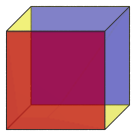Now the other faces of the cube are visible. We have colored the front face red, and the back face blue, and remaining faces yellow. The front face is connected to the back face by 4 edges.

For comparison, here is a 4D hypercube in orthographic projection: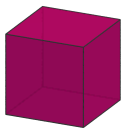Just as the image of the cube under orthographic projection is a 2D square, so the image of the hypercube in orthographic projection is a 3D cube.

Here's the 4D hypercube in oblique projection: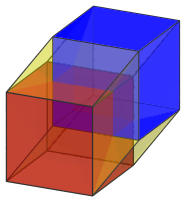We have colored the nearest cell of the hypercube red, and the farthest cell blue, and the remaining cells yellow. Note the similarity between this image and the oblique projection of the 3D cube. Just as the oblique projection of the cube looks like two squares connected by 4 lines, so the oblique projection of the hypercube looks like two cubes connected by 8 lines. You can see dimensional analogy at work here.

Some of the properties of images produced by parallel projection are:

• The size of the image does not depend on the distance of the object. No matter how far away the object is, the parallel rays will always produce the same image size.

• Parallel lines in the object remain parallel in the image. For example, in the oblique projection of the cube, the vertical edges of the cube all appear vertical in the image. The front-to-back edges appear slanted, but they are still parallel to each other, just as in the real cube.

Last updated 06 Feb 2018.